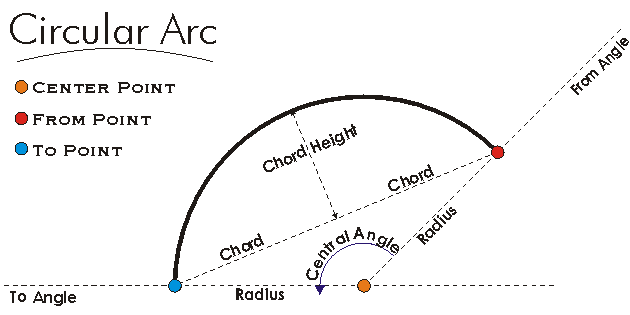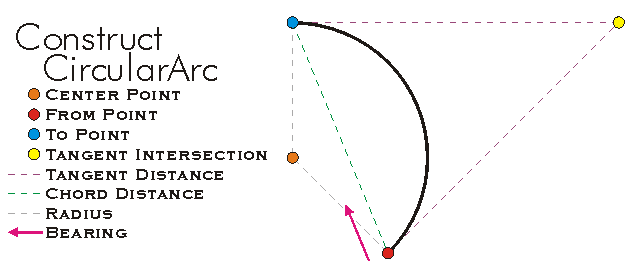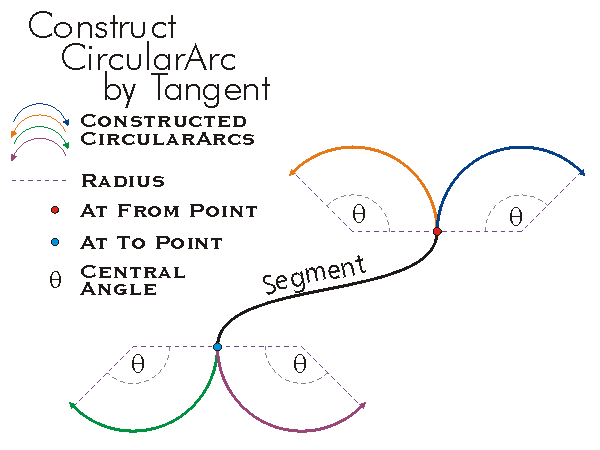ArcObjects Library Reference (Geometry)

# IConstructCircularArc Interface

Provides access to members that construct a circular arc using other geometries and values. Note: the IConstructCircularArc interface has been superseded byIConstructCircularArc2. Please consider using the more recent version.

#### Product Availability

Available with ArcGIS Engine, ArcGIS Desktop, and ArcGIS Server.

#### Description

IConstructCircularArc allows CircularArcs to be constructed given a wide variety of parameters.  The construction method varies depending on what parameters the user has available.  The input parameters used in the various constructions include the Center Point, the From Point, the To Point, a Thru Point, IsCounterClockwise Orientation, the Arc Distance, the Bearing Angle, the Central Angle, the Chord Distance, the Tangent Distance, the Radius, IsMinor Orientation, Fillet Segments, and a Tangent Segment.

The Central Angle used for construction must be positive (since the orientation is already specified).  The Arc Distance is the length of the CircularArc to be constructed.  The Bearing Angle is the Angle between the From Point and To Point of the CircularArc to be constructed.  The Chord Distance is the length of the chord between the From Point and To Point.  The Tangent Distance is the length of the line between an endpoint of the CircularArc to be constructed and the point of intersection between the embedded extension of the lines Tangent to the endpoints.  The Tangent Segment is the segment that determines an endpoint of the CircularArc to be constructed and the tangent at that endpoint.

Most construction methods use a single specified endpoint and a variety of parameters to calculate the other endpoint.  The calculated endpoint is rarely at the exact location of the theoretically calculated location based on small numerical inaccuracies in the computerized calculation process.  This is important to remember when attaching other segments to the constructed CircularArc.  ConstructThreePoints and the EndPoint construction methods always gives exact endpoints since they are both given as input.

#### Members

DescriptionConstructArcDistance Constructs an arc from a center point, a starting point, and an arc length.ConstructBearingAngleArc Constructs an arc with the given chord bearing, central angle and arc distance.ConstructBearingAngleChord Constructs an arc with the given chord bearing, central angle and chord distance.ConstructBearingAngleTangent Constructs an arc with the given chord bearing, central angle and tangent distance.ConstructBearingArcTangent Constructs an arc with the given chord bearing, arc distance and tangent distance.ConstructBearingChordArc Constructs an arc with the given chord bearing, chord distance and arc distance (negative for clockwise orientation).ConstructBearingChordTangent Constructs an arc with the given chord bearing, chord distance and tangent distance.ConstructBearingRadiusAngle Constructs an arc with the given chord bearing, radius and central angle.ConstructBearingRadiusArc Constructs an arc with the given chord bearing, radius and arc distance.ConstructBearingRadiusChord Constructs an arc with the given chord bearing, radius and chord distance.ConstructBearingRadiusTangent Constructs an arc with the given chord bearing, radius and tangent distance.ConstructChordDistance Constructs an arc from a center point, a starting point, and a chord length.ConstructCircle Constructs a circle of a given radius and orientation.ConstructEndPointsAngle Constructs an arc from the given endpoints and central angle.ConstructEndPointsArc Constructs an arc from the given endpoints and arc distance.ConstructEndPointsChordHeight Constructs an arc with specified endpoints and and chord height.ConstructEndPointsRadius Constructs an arc from the given endpoints and radius.ConstructEndPointsTangent Constructs an arc from the given endpoints and tangent distance.ConstructFilletPoint Constructs an arc of given start point near first segment and tangent to two segments. hintPoint can be nil or can be a location near the desired fillet.ConstructFilletRadius Constructs an arc of given radius and tangent to two segments. hintPoint can be nil or can be a location near the desired fillet.ConstructTangentAndPoint Constructs an arc with one endpoint being p, tangent to s, and connected to s. If atFrom is true, the connection is at s's from point, otherwise at s's to point.ConstructTangentAngleArc Constructs an arc with a common tangent to the input segment, a given central angle and an arc length.ConstructTangentAngleChord Constructs an arc with a common tangent to the input segment, a given central angle and a chord length.ConstructTangentAngleTangent Constructs an arc with a common tangent to the input segment, a given central angle and a tangent length.ConstructTangentArcTangent Constructs an arc with a common tangent to the input segment, a given arc length and a tangent length.ConstructTangentChordArc Constructs an arc with a common tangent to the input segment, a given chord length and an arc length.ConstructTangentChordTangent Constructs an arc with a common tangent to input segment, a given chord length and a tangent length.ConstructTangentDistance Constructs an arc from a center point, a starting point, and an tangent length.ConstructTangentRadiusAngle Constructs an arc with a common tangent to the input segment, a given radius and a central angle.ConstructTangentRadiusArc Constructs an arc having a common tangent to the input segment, a given radius and an arc length.ConstructTangentRadiusChord Constructs an arc with a common tangent to the input segment, a given radius and a chord length.ConstructTangentRadiusTangent Constructs an arc with a common tangent to the input segment, a given radius and a tangent length.ConstructThreePoints Constructs an arc from three points. useExistingCenter can be set to true in order to help create a reasonable arc when from and to are identical.QueryFilletRadiusRange Returns minimum and maximum radius for fillet to touch both input segments. hintPoint can be nil or can be a location near the desired fillet.

#### CoClasses that implement IConstructCircularArc

CoClasses and Classes Description
CircularArc A portion of a circle that connects two points optionally has measure, height and ID attributes at each endpoint.

#### Remarks